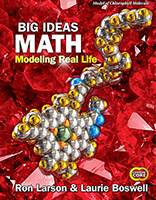1 Chapter 1 Adding and Subtracting Rational Numbers Section 1.1:  Rational Numbers Also consider: Section 1.1:  Rational Numbers Also consider: Section 1.2:  Adding Integers Also consider: Section 1.2:  Adding Integers Also consider: Section 1.3:  Adding Rational Numbers Section 1.3:  Adding Rational Numbers Section 1.4:  Subtracting Integers Also consider: Section 1.4:  Subtracting Integers Also consider: Section 1.5:  Subtracting Rational Numbers Also consider: Section 1.5:  Subtracting Rational Numbers Also consider:
 2 Chapter 2 Multiplying and Dividing Rational Numbers Section 2.1:  Multiplying Integers Section 2.1:  Multiplying Integers Section 2.2:  Dividing Integers Also consider: Section 2.2:  Dividing Integers Also consider: Section 2.3:  Converting Between Fractions and Decimals Also consider: Section 2.3:  Converting Between Fractions and Decimals Also consider: Section 2.4:  Multiplying Rational Numbers Section 2.4:  Multiplying Rational Numbers Section 2.5:  Dividing Rational Numbers Also consider: Section 2.5:  Dividing Rational Numbers Also consider:
 3 Chapter 3 Expressions Section 3.1:  Algebraic Expressions Also consider: Section 3.1:  Algebraic Expressions Also consider: Section 3.2:  Adding and Subtracting Linear Expressions Section 3.2:  Adding and Subtracting Linear Expressions Section 3.3:  The Distributive Property Also consider: Section 3.3:  The Distributive Property Also consider: Section 3.4:  Factoring Expressions Also consider: Section 3.4:  Factoring Expressions Also consider:
 4 Chapter 4 Equations and Inequalities Section 4.1:  Solving Equations Using Addition or Subtraction Section 4.1:  Solving Equations Using Addition or Subtraction Section 4.2:  Solving Equations Using Multiplication or Division Also consider: Section 4.2:  Solving Equations Using Multiplication or Division Also consider: Section 4.3:  Solving Two-Step Equations Also consider: Section 4.3:  Solving Two-Step Equations Also consider: Section 4.4:  Writing and Graphing Inequalities Also consider: Section 4.4:  Writing and Graphing Inequalities Also consider: Section 4.5:  Solving Inequalities Using Addition or Subtraction Section 4.5:  Solving Inequalities Using Addition or Subtraction Section 4.6:  Solving Inequalities Using Multiplication or Division Also consider: Section 4.6:  Solving Inequalities Using Multiplication or Division Also consider: Section 4.7:  Solving Two-Step Inequalities Section 4.7:  Solving Two-Step Inequalities
 5 Chapter 5 Ratios and Proportions Section 5.1:  Ratios and Ratio Tables Also consider: Section 5.1:  Ratios and Ratio Tables Also consider: Section 5.2:  Rates and Unit Rates Also consider: Section 5.2:  Rates and Unit Rates Also consider: Section 5.3:  Identifying Proportional Relationships Section 5.3:  Identifying Proportional Relationships Section 5.4:  Writing and Solving Proportions Section 5.4:  Writing and Solving Proportions Section 5.5:  Graphs of Proportional Relationships Also consider: Section 5.5:  Graphs of Proportional Relationships Also consider: Section 5.6:  Scale Drawings Also consider: Section 5.6:  Scale Drawings Also consider:
 6 Chapter 6 Percents Section 6.1:  Fractions, Decimals, and Percents Also consider: Section 6.1:  Fractions, Decimals, and Percents Also consider: Section 6.2:  The Percent Proportion Also consider: Section 6.2:  The Percent Proportion Also consider: Section 6.3:  The Percent Equation Section 6.3:  The Percent Equation Section 6.4:  Percents of Increase and Decrease Also consider: Section 6.4:  Percents of Increase and Decrease Also consider: Section 6.5:  Discounts and Markups Also consider: Section 6.5:  Discounts and Markups Also consider: Section 6.6:  Simple Interest Section 6.6:  Simple Interest
 7 Chapter 7 Probability Section 7.1:  Probability Also consider: Section 7.1:  Probability Also consider: Section 7.2:  Experimental and Theoretical Probability Also consider: Section 7.2:  Experimental and Theoretical Probability Also consider: Section 7.3:  Compound Events Also consider: Section 7.3:  Compound Events Also consider: Section 7.4:  Simulations Section 7.4:  Simulations
 8 Chapter 8 Statistics Section 8.1:  Samples and Populations Also consider: Section 8.1:  Samples and Populations Also consider: Section 8.2:  Using Random Samples to Describe Populations Section 8.2:  Using Random Samples to Describe Populations Section 8.3:  Comparing Populations • Coming soon: Make inferences about two populations Also consider: Section 8.3:  Comparing Populations • Coming soon: Make inferences about two populations Also consider: Section 8.4:  Using Random Samples to Compare Populations Section 8.4:  Using Random Samples to Compare Populations
 9 Chapter 9 Geometric Shapes Section 9.1:  Circles and Circumference Also consider: Section 9.1:  Circles and Circumference Also consider: Section 9.2:  Areas of Circles Section 9.2:  Areas of Circles Section 9.3:  Perimeters and Areas of Composite Figures Section 9.3:  Perimeters and Areas of Composite Figures Section 9.4:  Constructing Polygons Also consider: Section 9.4:  Constructing Polygons Also consider: Section 9.5:  Finding Unknown Angle Measures Section 9.5:  Finding Unknown Angle Measures
 10 Chapter 10 Surface Area and Volume Section 10.1:  Surface Area of Prisms Section 10.1:  Surface Area of Prisms Section 10.2:  Surface Area of Cylinders Section 10.2:  Surface Area of Cylinders Section 10.3:  Surface Area of Pyramids Also consider: Section 10.3:  Surface Area of Pyramids Also consider: Section 10.4:  Volumes of Prisms Section 10.4:  Volumes of Prisms Section 10.5:  Volumes of Pyramids Section 10.5:  Volumes of Pyramids Section 10.6:  Cross Sections of Three-Dimensional Figures Section 10.6:  Cross Sections of Three-Dimensional Figures
This document includes the IXL® skill alignments to Big Ideas Learning's Big Ideas Math 2019 curriculum. IXL provides skill alignments as a service to teachers, students, and parents. The skill alignments are provided by IXL and are not affiliated with, sponsored by, reviewed, approved or endorsed by Big Ideas Learning or any other third party. IXL® and IXL Learning® are registered trademarks of IXL Learning, Inc. All other intellectual property rights (e.g., unregistered and registered trademarks and copyrights) are the property of their respective owners.